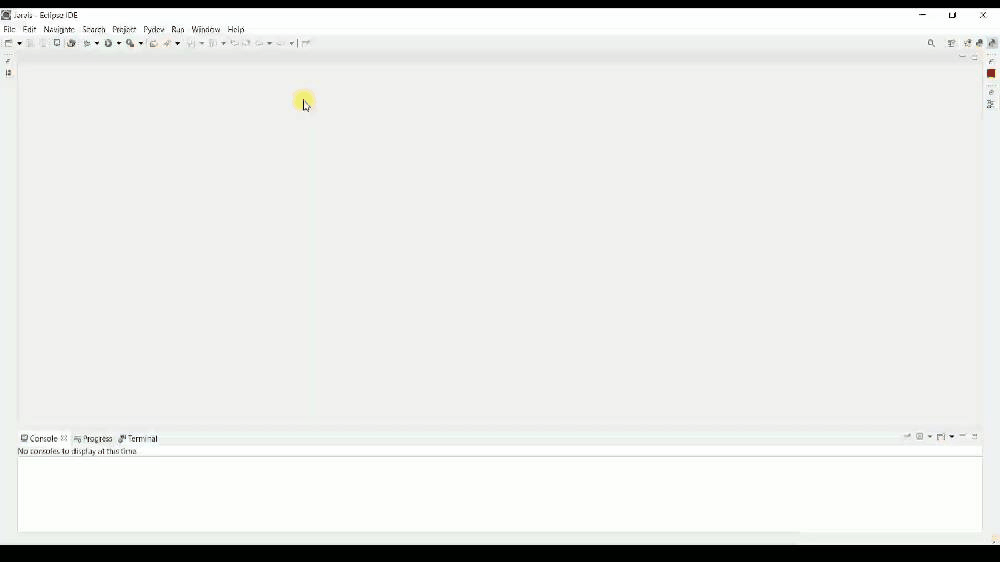1567652739

# How to code Gaussian Mixture Models from scratch in Python

They are parametric generative models that attempt to learn the true data distribution. Hence, once we learn the Gaussian parameters, we can generate data from the same distribution as the source.

We can think of GMMs as the soft generalization of the K-Means clustering algorithm. Like K-means, GMMs also demand the number of clusters K as an input to the learning algorithm. However, there is a key difference between the two. K-means can only learn clusters with a circular form. GMMs, on the other hand, can learn clusters with any elliptical shape.

Also, K-means only allows for an observation to belong to one, and only one cluster. Differently, GMMs give probabilities that relate each example with a given cluster. In other words, it allows for an observation to belong to more than one cluster — with a level of uncertainty. For each observation, GMMs learn the probabilities of that example to belong to each cluster k.

In general, GMMs try to learn each cluster as a different Gaussian distribution. It assumes the data is generated from a limited mixture of Gaussians.

Assuming one-dimensional data and the number of clusters K equals 3, GMMs attempt to learn 9 parameters.

• 3 parameters for the means
• 3 parameters for the variances
• 3 scaling parameters

Here, each cluster is represented by an individual Gaussian distribution (for this example, 3 in total). For each Gaussian, it learns one mean and one variance parameters from data. The 3 scaling parameters, 1 for each Gaussian, are only used for density estimation.

To learn such parameters, GMMs use the expectation-maximization (EM) algorithm to optimize the maximum likelihood. In the process, GMM uses Bayes Theorem to calculate the probability of a given observation xᵢ to belong to each clusters k, for k = 1,2,…, K.

Let’s dive into an example. For the sake of simplicity, let’s consider a synthesized 1-dimensional data. But, as we are going to see later, the algorithm is easily expanded to high dimensional data with D > 1. You can follow along using this jupyter notebook.

To build a toy dataset, we start by sampling points from K different Gaussian distributions. Each one (with its own mean and variance) represents a different cluster in our synthesized data. To make things clearer, let’s use K equals 2.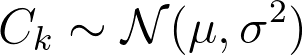Below, you can see the resulting synthesized data. We are going to use it as training data to learn these clusters (from data) using GMMs. Note that some of the values do overlap at some point.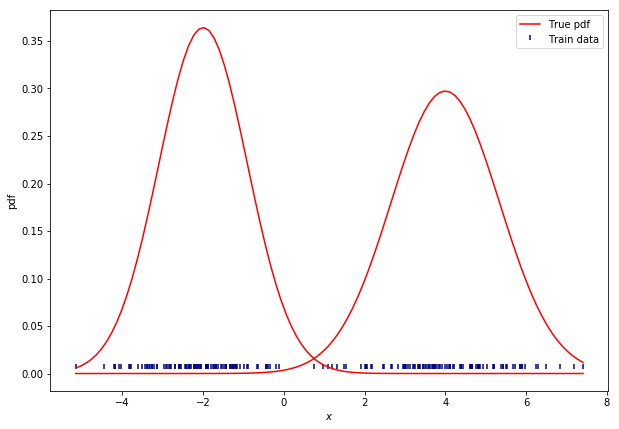We can think of GMMs as a weighted sum of Gaussian distributions. The number of clusters K defines the number of Gaussians we want to fit.

As we said, the number of clusters needs to be defined beforehand. For simplicity, let’s assume we know the number of clusters and define K as 2. In this situation, GMMs will try to learn 2 Gaussian distributions. For 1-dim data, we need to learn a mean and a variance parameter for each Gaussian.

Before we start running EM, we need to give initial values for the learnable parameters. We can guess the values for the means and variances, and initialize the weight parameters as 1/k.

Then, we can start maximum likelihood optimization using the EM algorithm. EM can be simplified in 2 phases: The E (expectation) and M (maximization) steps.

In the E step, we calculate the likelihood of each observation xᵢ using the estimated parameters.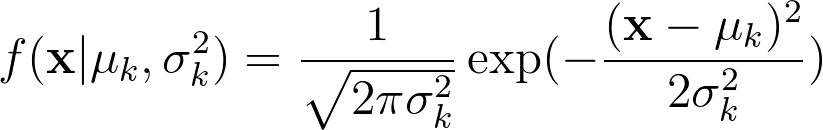1-d gaussian distribution equation

For each cluster k = 1,2,3,…,K, we calculate the probability density (pdf) of our data using the estimated values for the mean and variance. At this point, these values are mere random guesses.

Then, we can calculate the likelihood of a given example xᵢ to belong to the kᵗʰ cluster.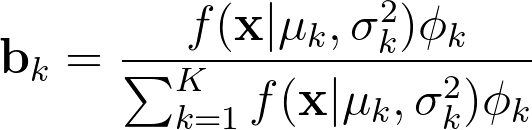Using Bayes Theorem, we get the posterior probability of the kth Gaussian to explain the data. That is the likelihood that the observation xᵢ was generated by kᵗʰ Gaussian. Note that the parameters Φ act as our prior beliefs that an example was drawn from one of the Gaussians we are modeling. Since we do not have any additional information to favor a Gaussian over the other, we start by guessing an equal probability that an example would come from each Gaussian. However, at each iteration, we refine our priors until convergence.

Then, in the maximization, or M step, we re-estimate our learning parameters as follows.Here, for each cluster, we update the mean (μₖ), variance (σ₂²), and the scaling parameters Φₖ. To update the mean, note that we weight each observation using the conditional probabilities bₖ.

We may repeat these steps until converge. That could be up to a point where parameters’ updates are smaller than a given tolerance threshold. At each iteration, we update our parameters so that it resembles the true data distribution.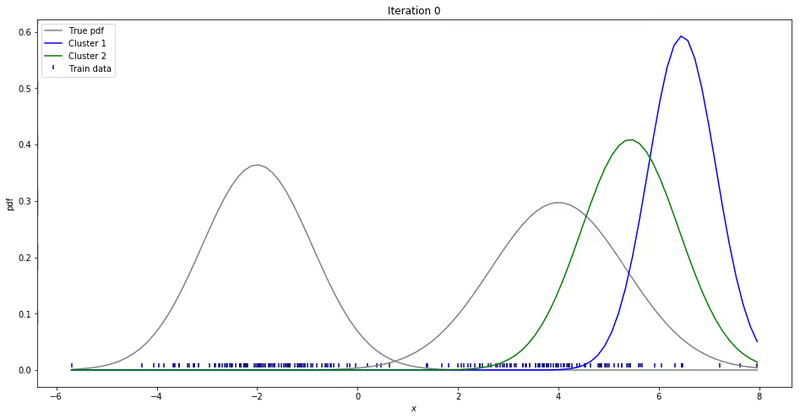Gaussian Mixture Models for 1D data using K equals 2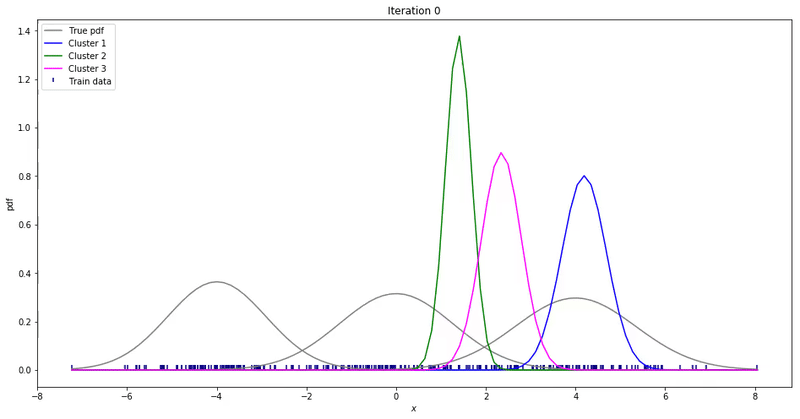For high-dimensional data (D>1), only a few things change. Instead of estimating the mean and variance for each Gaussian, now we estimate the mean and the covariance. The covariance is a squared matrix of shape (D, D) — where D represents the data dimensionality. Below, I show a different example where a 2-D dataset is used to fit a different number of mixture of Gaussians.

Check the jupyter notebook for 2-D data here.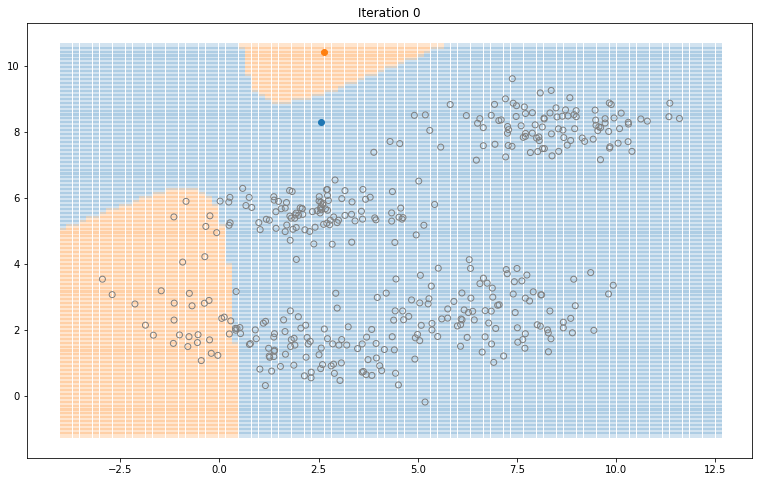Gaussian Mixture Models for 2D data using K equals 2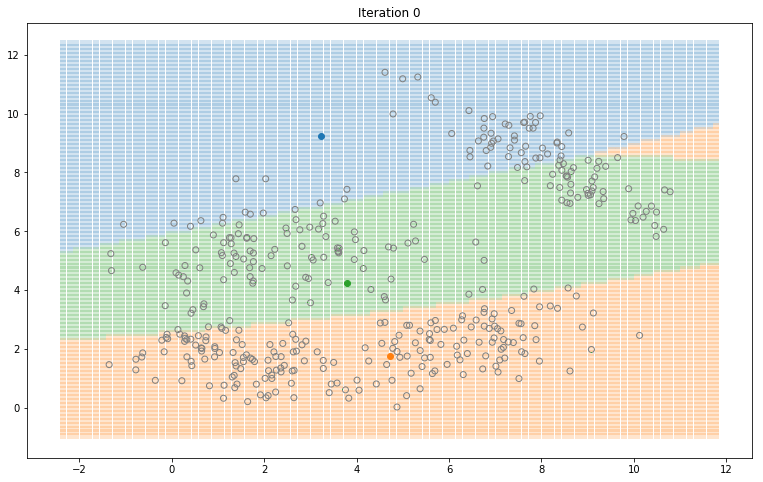Gaussian Mixture Models for 2D data using K equals 3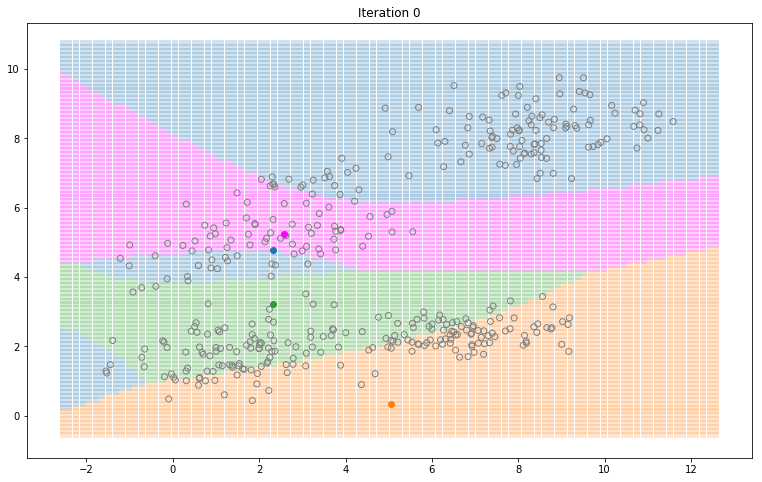Gaussian Mixture Models for 2D data using K equals 4

Note that the synthesized dataset above was drawn from 4 different gaussian distributions. Nevertheless, GMMs make a good case for twothree, and four different clusters.

That is it for Gaussian Mixture Models. These are some key points to take from this piece.

• GMMs are a family of generative parametric unsupervised models that attempt to cluster data using Gaussian distributions.
• Like K-Mean, you still need to define the number of clusters K you want to learn.
• Different from K-Means, GMMs represent clusters as probability distributions. This allows for one data points to belong to more than one cluster with a level of uncertainty.

============================================

#python #machine-learning #data-science

## Buddha Community1619518440

## top 30 Python Tips and Tricks for Beginners

Welcome to my Blog , In this article, you are going to learn the top 10 python tips and tricks.

### 8) Check The Memory Usage Of An Object.

#python #python hacks tricks #python learning tips #python programming tricks #python tips #python tips and tricks #python tips and tricks advanced #python tips and tricks for beginners #python tips tricks and techniques #python tutorial #tips and tricks in python #tips to learn python #top 30 python tips and tricks for beginners1619510796

## Lambda, Map, Filter functions in python

Welcome to my Blog, In this article, we will learn python lambda function, Map function, and filter function.

Lambda function in python: Lambda is a one line anonymous function and lambda takes any number of arguments but can only have one expression and python lambda syntax is

Syntax: x = lambda arguments : expression

Now i will show you some python lambda function examples:

#python #anonymous function python #filter function in python #lambda #lambda python 3 #map python #python filter #python filter lambda #python lambda #python lambda examples #python map1623077340

## 50+ Basic Python Code Examples

### List, strings, score calculation and more…

1. How to print “Hello World” on Python?

2. How to print “Hello + Username” with the user’s name on Python?

3. How to add 2 numbers entered on Python?

4. How to find the Average of 2 Entered Numbers on Python?

5. How to calculate the Entered Visa and Final Grade Average on Python?

6. How to find the Average of 3 Written Grades entered on Python?

7. How to show the Class Pass Status (PASSED — FAILED) of the Student whose Written Average Has Been Entered on Python?

8. How to find out if the entered number is odd or even on Python?

9. How to find out if the entered number is Positive, Negative, or 0 on Python?

#programming #python #coding #50+ basic python code examples #python programming examples #python code1626984360

## Common Anti-Patterns in Python

### 1. Not Using `with` to Open Files

When you open a file without the `with` statement, you need to remember closing the file via calling `close()` explicitly when finished with processing it. Even while explicitly closing the resource, there are chances of exceptions before the resource is actually released. This can cause inconsistencies, or lead the file to be corrupted. Opening a file via `with` implements the context manager protocol that releases the resource when execution is outside of the `with` block.

### 5. Not Using `get()` to Return Default Values From a Dictionary

#code reviews #python programming #debugger #code review tips #python coding #python code #code debugging1624238545

## Live Coding of Python in the Eclipse IDE

### This live coding extension makes coders/programmers life easier

Let’s get started…

In this article, I’ll be talking about how to use Live Coding features of Python in Eclipse.

Every time the programmer has to spend a lot of time debugging their code. And still, they failed to debug. This extension will help the coders or programmers to reduce their debugging time. This extension is downloadable in Eclipse IDE.

If you are unaware of how to install the extensions or plugins in eclipse.

• Open the Eclipse IDE and set up your workspace.
• Once done with the above steps, navigate to the **Help **menu tab.
• In the Help menu tab, click on the “Eclipse Marketplace” option.
• Search for **“Live Coding in Python” (**or use this link)andclick on the **Install **button.
• After click on the Install button, accept all the** terms and conditions.**